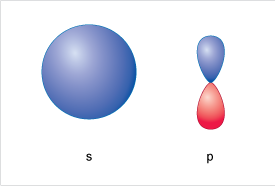Discovering chemistry

Start this free course now. Just create an account and sign in. Enrol and complete the course for a free statement of participation or digital badge if available.

Free course

# 1.10 More about atomic orbitals

Up to this point you’ve considered electrons as being arranged in a series of shells and sub-shells.

These sub shells can be described as specific locations within an energy level (shell) where there is a high probability of finding an electron.

Does this seem familiar?

Hopefully so, these are the atomic orbitals introduced in section 1.5, i.e. regions in space where there is a high probability of finding an electron.

A representation of an s-orbital and a p orbital are shown in Figure 8.Figure 8 Representation of an s and a p orbital

So this means each shell will have at least one orbital associated with it.

Using this idea, let’s now try and build up a more detailed picture of the atom.

Starting at lowest energy shell (n = 1), closest to the nucleus you will find a 1s orbital – this is spherical.

At higher energy, and further from the nucleus in the n=2 shell, you will find two kinds of orbital 2s and 2p. The 2s is also spherical as per the 1s but is (rather obviously) labelled 2 to represent the higher energy shell, and the larger radius than the 1s.

Moving to the n = 3 shell you find d-orbitals, and in the n=4 shell f-orbitals appear, these won’t be considered here.

DC_1